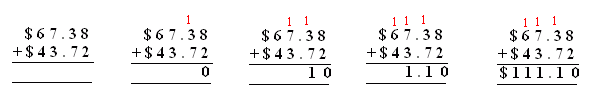Name: ___________________Date:___________________

 Email us to get an instant 20% discount on highly effective K-12 Math & English kwizNET Programs!

 Method The numbers after the decimal point are read as tenths, hundreths and so on. The numbers before the decimal point are read as hundreds, tens and ones. Start adding from hundreths place. Move on to the tenths, tens, ones and so on. Example:Start adding from the right most number after the decimal point. The result is sum of 6 and 2 which is 8. The next is sum of 5 and 1 which gives 6. The result to the left of decimal is sum of 4 and 2 which is 6. The next is sum of 3 and 3 which is 6. Answer: 66.68 Example:Start adding from the right most number after the decimal point. The result is sum of 8 and 2 which is 10. This results in a carry of 1 to the next digit. The next is sum of 3 and 7 and the carry 1. The result is 11. The next is sum of 7 and 3 and a carry 1. The result is 11. The next is sum of 6 and 4 with a carry 1. The result is 11. Answer: 111.10 Directions: Adding the following dollars and cents. Also write at least ten examples of your own.
 Question 1: \$ 33.05 + \$ 25.61

 Question 2: \$ 91.41 + \$ 23.81

 Question 3: \$ 67.85 + \$ 20.06

 Question 4: \$ 89.68 + \$ 73.57

 Question 5: \$ 44.28 + \$ 53.11

Question 6: This question is available to subscribers only!

Question 7: This question is available to subscribers only!

Question 8: This question is available to subscribers only!

Question 9: This question is available to subscribers only!

Question 10: This question is available to subscribers only!